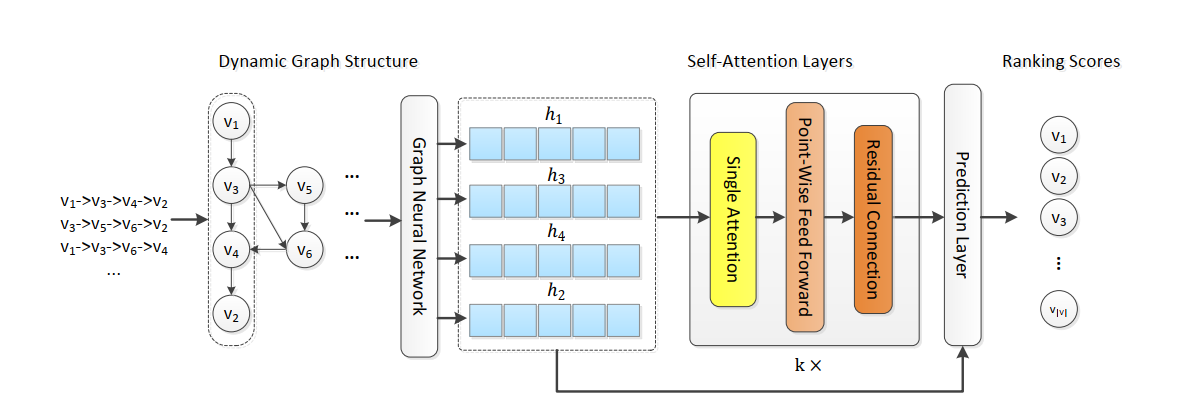# GCSAN¶

## Introduction¶

[paper]

Title: Graph Contextualized Self-Attention Network for Session-based Recommendation

Authors: Chengfeng Xu, Pengpeng Zhao, Yanchi Liu, Victor S. Sheng, Jiajie Xu, Fuzhen Zhuang, Junhua Fang, Xiaofang Zhou

Abstract: Session-based recommendation, which aims to predict the user’s immediate next action based on anonymous sessions, is a key task in many online services (e:g:; e-commerce, media streaming). Recently, Self-Attention Network (SAN) has achieved significant success in various sequence modeling tasks without using either recurrent or convolutional network. However, SAN lacks local dependencies that exist over adjacent items and limits its capacity for learning contextualized representations of items in sequences. In this paper, we propose a graph contextualized self-attention model (GC-SAN), which utilizes both graph neural network and self-attention mechanism, for session-based recommendation. In GC-SAN, we dynamically construct a graph structure for session sequences and capture rich local dependencies via graph neural network (GNN). Then each session learns long-range dependencies by applying the self-attention mechanism. Finally, each session is represented as a linear combination of the global preference and the current interest of that session. Extensive experiments on two real-world datasets show that GC-SAN outperforms state-of-the-art methods consistently.## Running with RecBole¶

Model Hyper-Parameters:

• `hidden_size (int)` : The number of features in the hidden state. It is also the initial embedding size of item. Defaults to `64`.

• `inner_size (int)` : The inner hidden size in feed-forward layer. Defaults to `256`.

• `n_layers (int)` : The number of transformer layers in transformer encoder. Defaults to `2`.

• `n_heads (int)` : The number of attention heads for multi-head attention layer. Defaults to `2`.

• `hidden_dropout_prob (float)` : The probability of an element to be zeroed. Defaults to `0.5`.

• `attn_dropout_prob (float)` : The probability of an attention score to be zeroed. Defaults to `0.5`.

• `hidden_act (str)` : The activation function in feed-forward layer. Defaults to `'gelu'`. Range in `['gelu', 'relu', 'swish', 'tanh', 'sigmoid']`.

• `layer_norm_eps (float)` : A value added to the denominator for numerical stability. Defaults to `1e-12`.

• `initializer_range (float)` : The standard deviation for normal initialization. Defaults to `0.02`.

• `step (int)` : The number of layers in GNN. Defaults to `1`.

• `weight (float)` : The weight parameter controls the contribution of self-attention representation and the last-clicked action, the original paper suggests that setting w to a value of 0.4 to 0.8 is more desirable. Defaults to `0.6`.

• `reg_weight (float)` : The L2 regularization weight. Defaults to `[5e-5]`.

• `loss_type (str)` : The type of loss function. If it is set to `'CE'`, the training task is regarded as a multi-classification task and the target item is the ground truth. In this way, negative sampling is not needed. If it is set to `'BPR'`, the training task will be optimized in the pair-wise way, which maximizes the difference between the positive item and the negative one. In this way, negative sampling is necessary, such as setting `--train_neg_sample_args="{'distribution': 'uniform', 'sample_num': 1}"`. Defaults to `'CE'`. Range in `['BPR', 'CE']`.

A Running Example:

Write the following code to a python file, such as run.py

```from recbole.quick_start import run_recbole

parameter_dict = {
'train_neg_sample_args': None,
}
run_recbole(model='GCSAN', dataset='ml-100k', config_dict=parameter_dict)
```

And then:

```python run.py
```

## Tuning Hyper Parameters¶

If you want to use `HyperTuning` to tune hyper parameters of this model, you can copy the following settings and name it as `hyper.test`.

```learning_rate choice [0.01,0.005,0.001,0.0005,0.0001]
step choice 
n_layers choice 
hidden_size choice 
inner_size choice 
hidden_dropout_prob choice [0.2]
attn_dropout_prob choice [0.2]
hidden_act choice ['gelu']
layer_norm_eps choice [1e-12]
initializer_range choice [0.02]
weight choice [0.5,0.6]
reg_weight choice [5e-5]
```

Note that we just provide these hyper parameter ranges for reference only, and we can not guarantee that they are the optimal range of this model.

Then, with the source code of RecBole (you can download it from GitHub), you can run the `run_hyper.py` to tuning:

```python run_hyper.py --model=[model_name] --dataset=[dataset_name] --config_files=[config_files_path] --params_file=hyper.test
```

For more details about Parameter Tuning, refer to Parameter Tuning.

If you want to change parameters, dataset or evaluation settings, take a look at